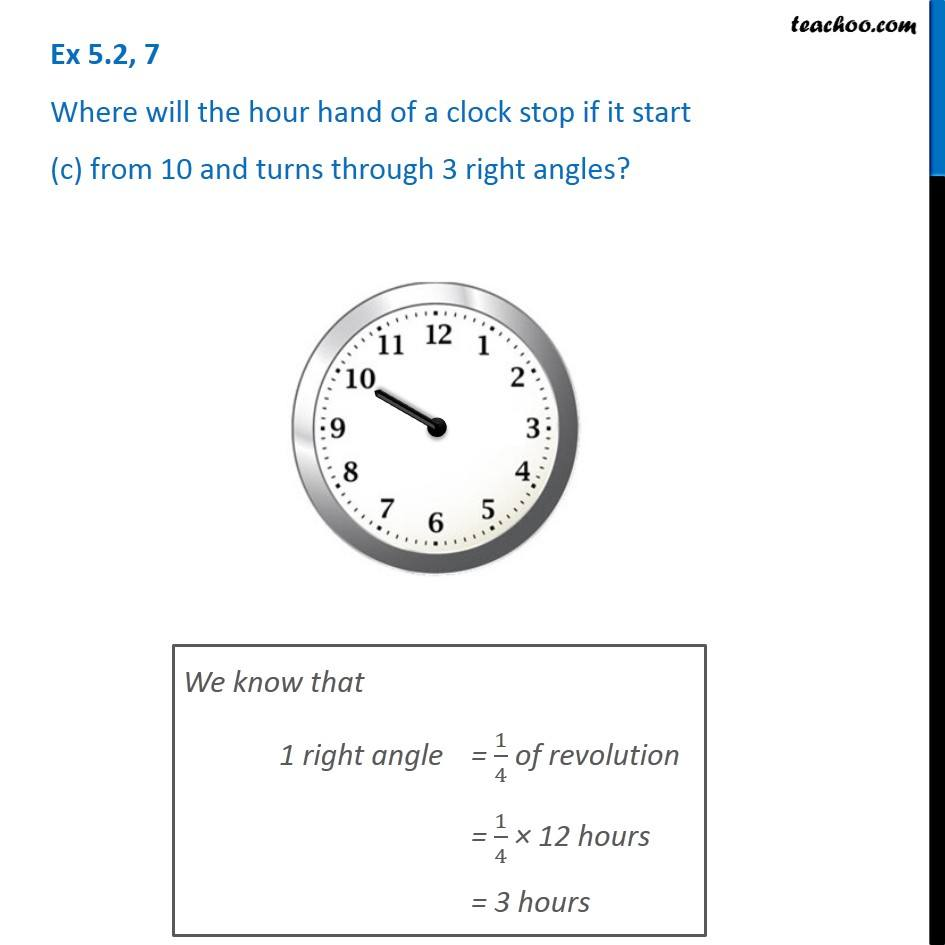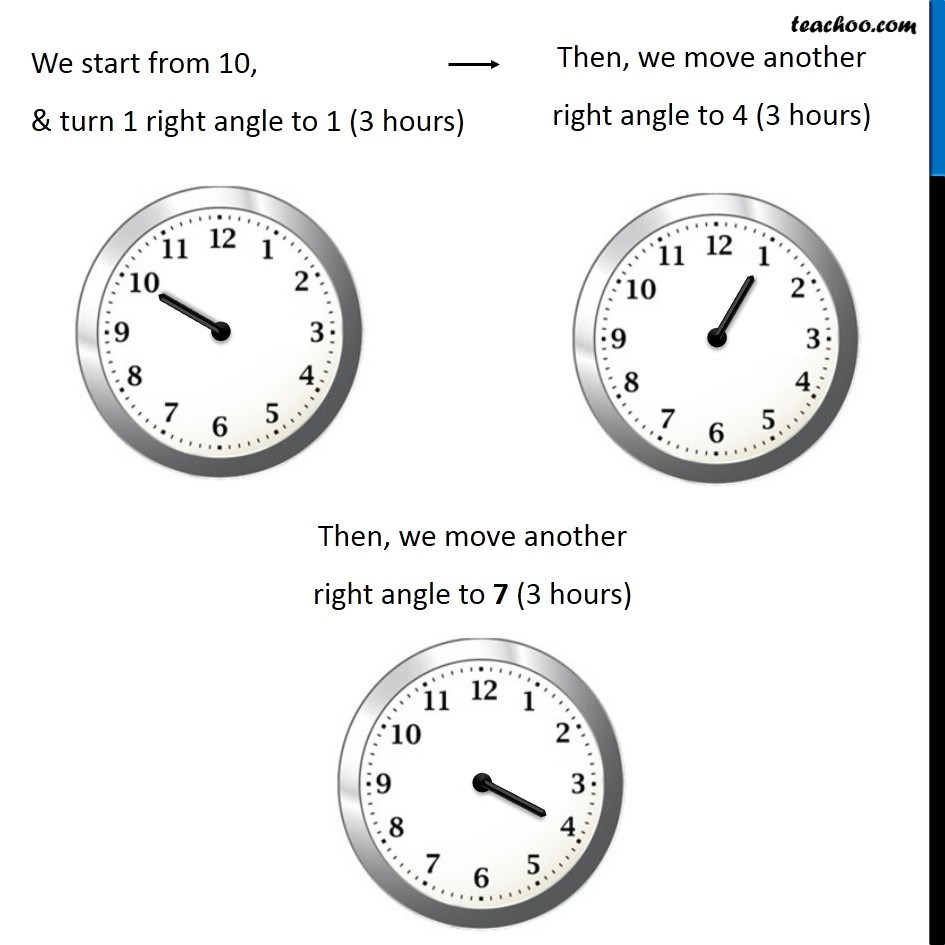1. Chapter 5 Class 6 Understanding Elementary Shapes
2. Serial order wise
3. Ex 5.2

Transcript

Ex 5.2, 7 Where will the hour hand of a clock stop if it start (c) from 10 and turns through 3 right angles? We know that 1 right angle = 1/4 of revolution = 1/4 × 12 hours = 3 hours We start from 10, & turn 1 right angle to 1 (3 hours) Then, we move another right angle to 4 (3 hours) Then, we move another right angle to 7 (3 hours)